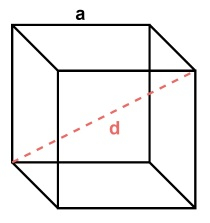# C++ Program to calculate the volume of Cube

Cubes are the basic 3D objects which have 8 vertices, 12-edges, and 6-faces. The volume of a 3D object is how much space it is occupying in the world. In this article, we will see how we can calculate the volume of a cube by writing a C++ program.

A cube has all edges of equal length. The area for each face is 𝑘2 where 𝑘 is the length of each side. As 3D objects have length, breadth, and width, so the volume of it will be 𝑘3. Let us see the algorithm and corresponding C++ implementation to find the volume of a cube object.

## Algorithm

• Take the length of the side of a cube, say k.
• Volume := k^3.
• Return Volume.

## Example

#include <iostream>
using namespace std;

int solve( int k ) {
int volume;
volume = k * k * k;
return volume;
}
int main() {
cout << "Volume of a cube with side length k = 5 cm, is " <<
solve( 5 ) << " cm^3" << endl;
cout << "Volume of a cube with side length k = 2 m, is " << solve( 2 ) << " m^3" << endl;
cout << "Volume of a cube with side length k = 25 in, is " <<
solve( 25 ) << " in^3" << endl;
}


## Output

Volume of a cube with side length k = 5 cm, is 125 cm^3
Volume of a cube with side length k = 2 m, is 8 m^3
Volume of a cube with side length k = 25 in, is 15625 in^3


A cube volume can also be calculated using its diagonal. The diagonal is not the same as the diagonal of its faces. The cube-diagonal is the straight line between two distance corners of the opposite side and passes through the center of the cube. See the following diagram for a better understanding.Here each side length is ‘a’ and the diagonal length is ‘d’. From the side length, we can calculate the length of the diagonal which is −

$$d\:=\:\sqrt{3}\:*\:a$$

And when the value of 𝑑 is known, we can calculate the volume with a simple formula, like below −

$$Volume\:=\:\sqrt{3}\:*\frac{d^3}{9}$$

This formula is quite straightforward. If we replace $d\:=\:\sqrt{3}\:*\:a$ here, the formula becomes.

$$Volume\:=\:\sqrt3*\frac{(\sqrt3*\:a)^3}{9}$$

$$Volume\:=\:\sqrt3*\frac{3\sqrt3*\:a^3}{9}$$

$$Volume\:=\:\frac{9a^3}{9}$$

$$Volume\:=\:a^3$$

Which is nothing but the previous formula that we have seen.

Now let us see the C++ implementation of this formula where we take the diagonal of a cube as input, and calculate the volume by using this above-mentioned formula.

## Algorithm

• Take the diagonal of the cube d.
• $Volume\:=\:\sqrt{3}\:*\frac{d^3}{9}$.

• Return Volume.

## Example

#include <iostream>
#include <cmath>

using namespace std;
float solve( float d ) {
float volume;
volume = sqrt(3) * (d * d * d) / 9;
return volume;
}
int main(){
cout << "Volume of a cube with diagonal length d = 1.732 cm, is " << solve( 1.732 ) << " cm^3" << endl;
cout << "Volume of a cube with diagonal length d = 5 cm, is " << solve( 5 ) << " cm^3" << endl;
cout << "Volume of a cube with diagonal length d = 2.51 cm, is "  << solve( 2.51 ) << " cm^3" << endl;
}


## Output

Volume of a cube with diagonal length d = 1.732 cm, is 0.999912 cm^3
Volume of a cube with diagonal length d = 5 cm, is 24.0563 cm^3
Volume of a cube with diagonal length d = 2.51 cm, is 3.04326 cm^3


## Conclusion

Calculating the volume of a cube is a very trivial process where we perform a cube (raised to the power 3) of the length of the side of the cube. Sometimes we may not know the length of the sides of a cube, but if we know the diagonal length, the cube volume can also be calculated. We have discussed these two methods to find the volume of a cube. To use the diagonal, in C++ we need to use the square-root operation which can be done by calling the sqrt() method present inside the cmath library. We can also perform a cube using the pow() function. But here we have multiplied the value three times to calculate the cube.

Updated on: 17-Oct-2022

2K+ Views